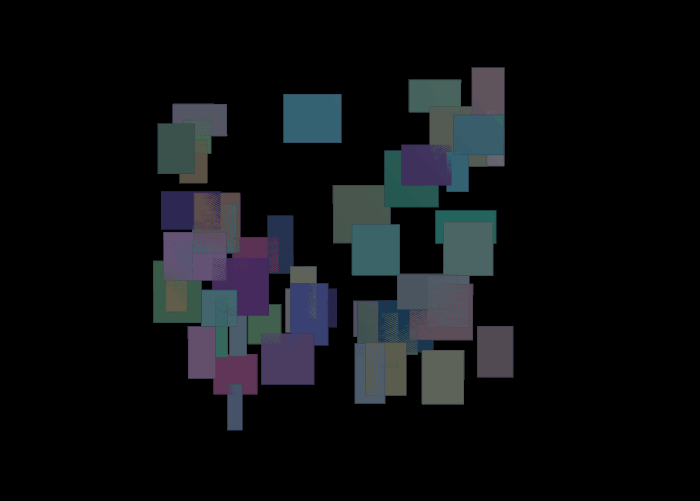## Recommended Posts

Posted (edited)

Currently wondering if it's possible to do something similar to the image below:

I want to be able to pack rectangles and squares into a uneven grid of points, 2x2, 2x4, 1x3, etc.. I'm not sure how to go about this those as it doesn't seem as simple as making a 2d array and checking if a pointer goes out of bounds?

I don't mind if it's brute forced as all the squares don't need to be filled, just most of them. Struggling to find a solution to this though

Edited by Pidgy

##### Share on other sites

The points in your image still appear to be a regular square grid with irregular edges, so you could probably accomplish what you are looking for using a grid and some VEX to delete prims that you don't want.  I would probably delete them randomly in a while loop, then come up with a check that would be able to detect the patterns.  I will have to noodle on it, though I do have some ideas.  I'm thinking using area vs perimeter to check if a block has become a valid shape since there is a unique set for both that will mean it has the right shape.  That only works if you have a uniform area per prim though.  If you want to use non-uniform, it gets much more complex.  Doing it this way could actually allow you to select any shape, though mirrors would be identical so an L shape could be possible to find, but the other 3 variations of it would pop up as well.  You could also have a half-edge calculation walk around the shape and check the number of corners, so any 4 corner shape would indicate that it is a rectangle, anything more would be ignored.

No matter how you slice it, you are asking about a very interesting Comp Sci/Math problem.

##### Share on other sites
Posted (edited)

Unless I'm misunderstanding, this seems to essentially be a shape/object packing problem - there have been threads on that here with a number of example files, so if that is indeed what you're after, I'm sure you'll find a solution previously posted

Plus an entagma tutorial you might find useful:

Edited by ryew

##### Share on other sites

It is completely a packing problem, I was just trying to keep some of the math speak out of my response.  I will definitely look at that post you linked to, looks juciy.

##### Share on other sites
Posted (edited)

Fun
points Grid

```vector2 pos = rand (@elemnum)-{.5,.5};
pos *= 10;

vector scale = fit01(rand(@elemnum+0.1),{0.2,0.5,1},{1,1,1});

float pscale = length(set(scale.x,scale.y));
setpointattrib(0,"scale",pt,scale);
setpointattrib(0,"pscale",pt,pscale);

vector nor= {0,0,1};

vector up = fit01(rand(@elemnum*0.2),{-1,-1,0},{1,1,0});
up= normalize(up);
setpointattrib(0,"up",0,up);```

solver

```removevalue(near_pts,@ptnum);

vector avg;
vector scale;
vector pos;
vector dir;
vector up;

int n = len(near_pts);
foreach(int pt; near_pts){
scale = point(0,"scale",pt);
pos = point (0,"P",pt);

dir = @P-pos;
up = point(0,"up",pt);

if(abs(dot(up,@up))>0.001){
@up += length(@up-up)*(up-@up)/n;
}

if(abs(dot(dir,v@side))<scale.x+@scale.x && abs(dot(dir,@up))<scale.y+@scale.y){
avg += 1.2*(@P-pos)/pow(length(@P-pos),4);

} else if(n<chi("compactnes")){
avg += pow(length(dir),2)*(pos-@P)/n;
}
}

@P += 0.008*normalize(avg);```

outside

```int pts[];
matrix xform = instance(@P,@N,@scale,0,@up);

foreach (vector pos;{{-1,-1},{-1,1},{1,1},{1,-1}}){

pos *= xform;
}

setprimattrib(0,"Cd",prim,vector(rand(0.1*@ptnum)));

removepoint(0,@ptnum);
```Edited by Librarian
•1

##### Share on other sites

That is a really interesting solution and I like it.  I would like to figure out how to make it minimized though, so there is a minimum total gap space.

## Create an account

Register a new account